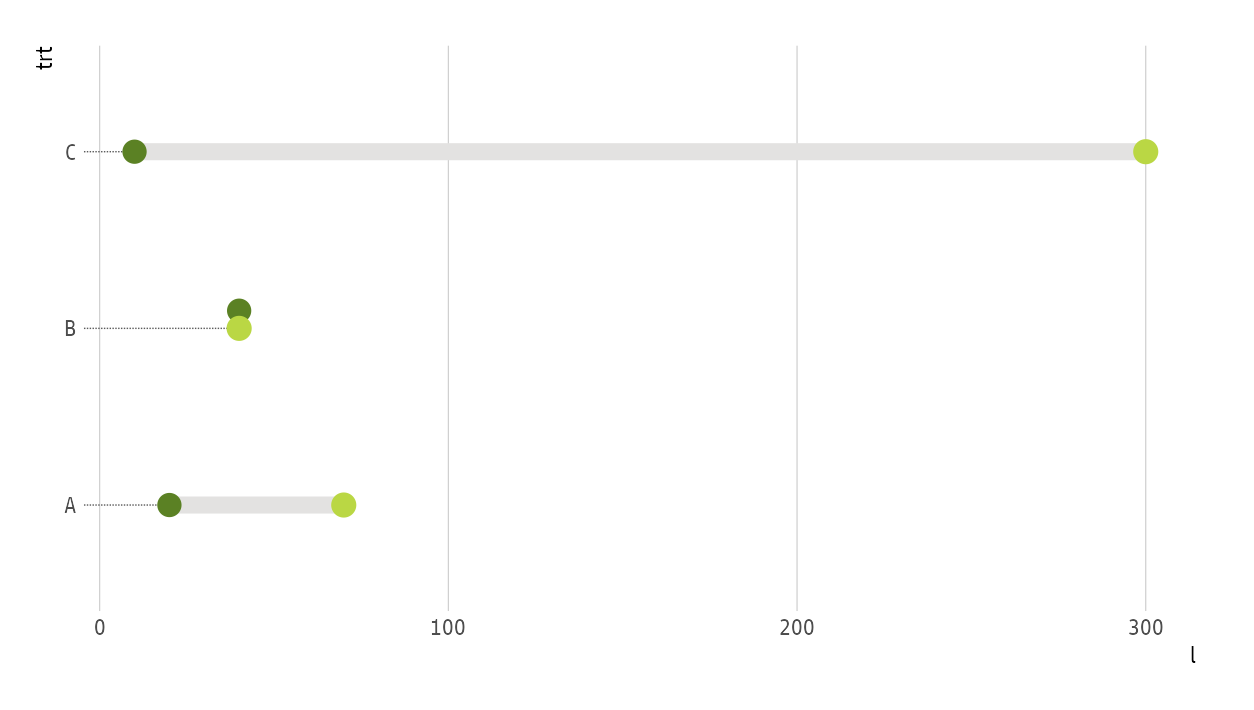# Make Multi-point “dumbbell” Plots in ggplot2

A user of the `{ggalt}` package recently posted a question about how to add points to a `geom_dumbbell()` plot. For now, this is not something you can do with `geom_dumbbell()` but with a bit of data wrangling you can do this in a pretty straightforward manner with just your data and ggplot2. The example below uses 3 values per category but it should scale to `n` values per category (though after a certain `n` you should reconsider the use of a dummbell chart in favour of a more appropriate way to visualize the message you’re trying to convey).

Here’s the setup:

``````library(hrbrthemes)
library(tidyverse)

tibble(
val1 = c(3, 2, 4),
val2 = c(1, 4, 5),
val3 = c(5, 8, 6),
cat = factor(month.name[1:3], levels = rev(month.name[1:3]))
) -> xdf
``````

Three values per category. The approach is pretty straightforward:

• reshape the data frame & get min value so you can draw an eye-tracking line (this is one geom)
• reshape the data frame & get min/max category values so you can draw the segment (this is another geom)
• reshape the data frame & plot the points

I’ve put ^^ notes near each ggplot2 geom:

``````ggplot() +
# reshape the data frame & get min value so you can draw an eye-tracking line (this is one geom)
geom_segment(
data = gather(xdf, measure, val, -cat) %>%
group_by(cat) %>%
top_n(-1) %>%
slice(1) %>%
ungroup(),
aes(x = 0, xend = val, y = cat, yend = cat),
linetype = "dotted", size = 0.5, color = "gray80"
) +
# reshape the data frame & get min/max category values so you can draw the segment (this is another geom)
geom_segment(
data = gather(xdf, measure, val, -cat) %>%
group_by(cat) %>%
summarise(start = range(val), end = range(val)) %>%
ungroup(),
aes(x = start, xend = end, y = cat, yend = cat),
color = "gray80", size = 2
) +
# reshape the data frame & plot the points
geom_point(
data = gather(xdf, measure, value, -cat),
aes(value, cat, color = measure),
size = 4
) +
# i just extended the scale a bit + put axis on top; choose aesthetics that work
# for you
scale_x_comma(position = "top", limits = c(0, 10)) +
scale_color_ipsum(name = "A real legend title") +
labs(
x = "Description of the value", y = NULL,
title = "A good plot title"
) +
theme_ipsum_rc(grid = "X") +
theme(legend.position = "top")
``````

And, here’s the result:Amazon Author Page

## 7 Comments →Make Multi-point “dumbbell” Plots in ggplot2

1.numerategeek

thanks very much for this. very helpful and timely – I was thinking of using dumbbell charts to visualize some of my data. thank you!

2.Peet

Hey Thanks for the post I have follow-up question is there a way to address the issue of two values that are the same size? I used this method and it worked great except in a situation where I have the same value. Is it possible to stack the point in such a way that they don’t completely overlap? Thank you

1.hrbrmstr

I should be able to add a horizontal jitter argument w/o too much effort.

2.hrbrmstr

This took a bit longer than expected. There’s a new “position” function in the `noproj` branch of {ggalt} called `position_jitter_on_equal` that has an `amount` parameter that takes a value between 0 and 1. Set the `position` parameter of `geom_dumbbell` to this new function and it will jitter the second dot’s y value by the amount specified. An example is below.

I’ve only tested it lightly, but it appears to work consistently.

Let me know how it goes and I’ll get it on CRAN at some point in early 2021.

``````data.frame(
trt = c(LETTERS[1:3]),
l = c(20, 40, 10),
r = c(70, 40, 300)
) -> df3

ggplot(df3, aes(y = trt, x = l, xend = r)) +
geom_dumbbell(
size = 3, color = "#e3e2e1",
colour_x = "#5b8124", colour_xend = "#bad744",
dot_guide = TRUE, dot_guide_size = 0.25,
position = position_jitter_on_equal(amount = 0.05)
) +
hrbrthemes::theme_ipsum_gs(grid="X")
``````This site uses Akismet to reduce spam. Learn how your comment data is processed.